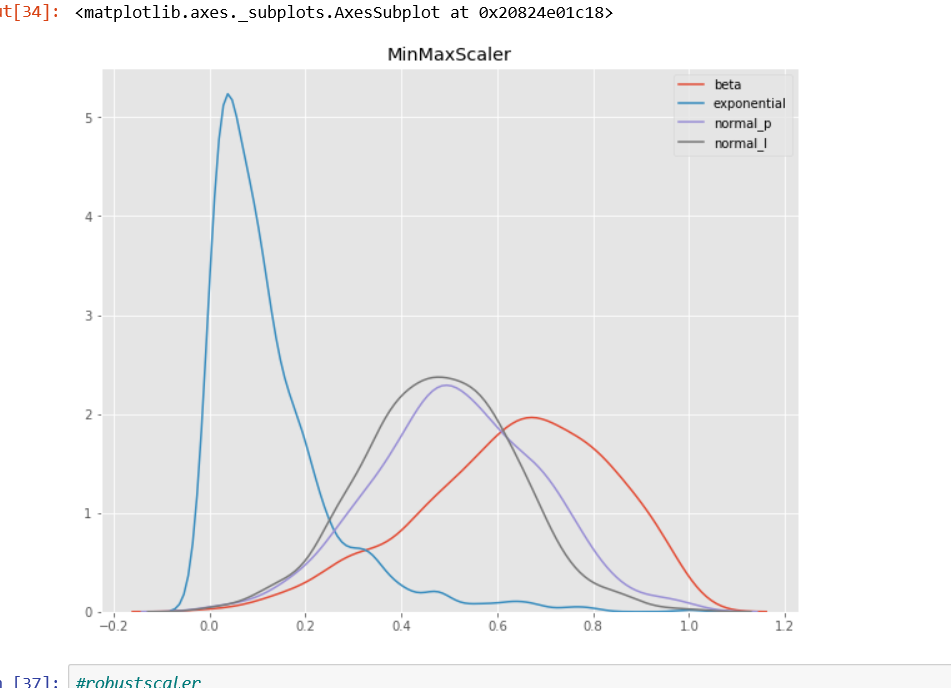# Why Scaling is Important in Machine Learning?

Source: Deep Learning on Medium# Why Scaling is Important in Machine Learning?

Ml algorithm works better when features are relatively on a similar scale and close to Normal Distribution.

## Let us understand the meaning of SCALE, STANDARDIZE AND NORMALIZE

1. SCALE– It means to change the range of values but without changing the shape of distribution. Range is often set to 0 to 1.
2. STANDARDIZE-It means changing values so that distribution standard deviation from mean equals to one,output will be very close to normal distribution.
3. NORMALIZE-It can be used either of above things, it can be a confusion to use this word so i personally do not use this often.

## WHY DO WE NEED TO STANDARDIZE OR NORMALIZE OUR FEATURE?

Algorithm converge faster when features are relatively smaller or closer to normal distribution.

Example of such algorithms are:

1. Linear and Logistic Regression
2. k nearest neighbor
3. Neural Network
4. PCA
5. LDA
6. SVM with radial bias kernel function

## Scikit-Learn library gives us some good options to scale or normalize our features.

1. MinMaxScaler()
2. RobustScaler()
3. StandardScaler()
4. Normalizer()

Lets us do the small python project to understand these scalers.

## Original Data

I created four distributions with different characteristics. The distributions are:

• beta — with negative skew
• exponential — with positive skew
• normal_p — normal
• normal_l — normal

The values all are of relatively similar scale, as can be seen on the X axis of the Kernel Density Estimate plot (kdeplot) below.

## MinMaxScaler

MinMaxScaler subtracts the minimum value in the feature and then divides by the range. The range is the difference between the original maximum and original minimum.

MinMaxScaler preserves the shape of the original distribution. It doesn’t meaningfully change the information embedded in the original data.

Note that MinMaxScaler doesn’t reduce the importance of outliers.

The default range for the feature returned by MinMaxScaler is 0 to 1.

Here’s the kdeplot after MinMaxScaler has been applied.

## RobustScaler

RobustScaler transforms the feature vector by subtracting the median and then dividing by the interquartile range (75% value — 25% value).

Note that RobustScaler does not scale the data into a predetermined interval like MinMaxScaler. It does not meet the strict definition of scale I introduced earlier.

Note that the range for each feature after RobustScaler is applied is larger than it was for MinMaxScaler.

Use RobustScaler if you want to reduce the effects of outliers, relative to MinMaxScaler.

## StandardScaler

StandardScaler standardizes a feature by subtracting the mean and then scaling to unit variance. Unit variance means dividing all the values by the standard deviation. StandardScaler does not meet the strict definition of scale I introduced earlier.

StandardScaler results in a distribution with a standard deviation equal to 1. The variance is equal to 1 also, because variance = standard deviation squared. And 1 squared = 1.

StandardScaler makes the mean of the distribution 0. About 68% of the values will lie be between -1 and 1.

## Normalizer

Normalizer works on the rows, not the columns! I find that very unintuitive. It’s easy to miss this information in the docs.

By default, L2 normalization is applied to each observation so the that the values in a row have a unit norm. Unit norm with L2 means that if each element were squared and summed, the total would equal 1. Alternatively, L1 (aka taxicab or Manhattan) normalization can be applied instead of L2 normalization.

## CONCLUSION

1. Use MinMaxScaler() if you are transforming a feature, its non distorting
2. Use RobustScaler() if you have outliers, this scaler will reduce the effect the influece of outliers
3. Use StandardScaler for relatively Normal Distribution
4. I don’t know what is best case to use normalize, if any one of the readers know, please comment.